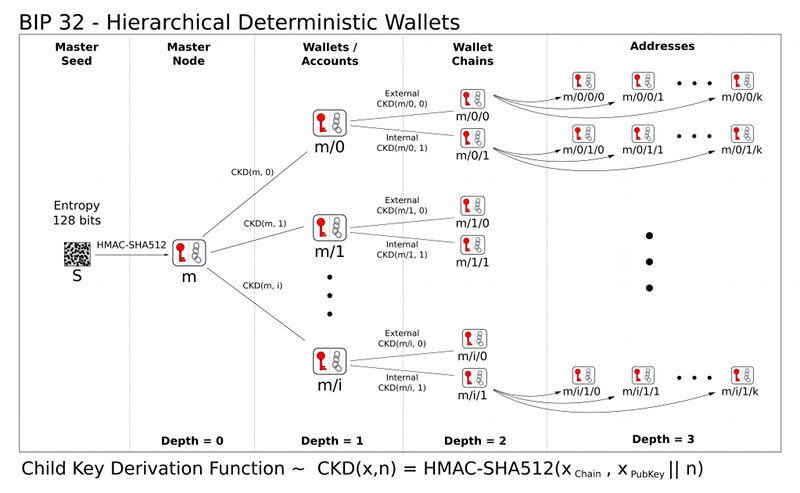# Note on Bip32

wiki

The specification consists of two parts:

1. a system for deriving a tree of keypairs from a single seed.

2. demostrate how to build a wallet structure on top of such a tree.

# Specification: Key derivation

## Convention

In this text we assume the public key cryptography used in Bitcoin, namely elliptic curve cryttography using the field and curve parameters defined by secp256k1. Variables below are either:

• Integers modulo the order of the curve (referred to as n)

• Coordinates of points on the curve

• Byte sequences

Addition (+) of two coordinate pair is defined as application of the EC group operation. Concatenation(||) is the operation of appending one byte sequence onto another.

## Extended Keys

We are going to define a function to derive a number of child keys from a parent key. In order to prevent these from depending solely on the key itself, we extend both private key and public key first with an extra 256 bits of entropy. This extention, called the chain code, is identical for corresponding private key and public keys, and consist of 256 bits, namely 32 bytes.

We represent an extended private key as (k, c), with k the normal private key and the c, the chain code. And extended public key is represented as (K, c), with K = point(k) and the c the chain code.

Each extended key has 2^31 normal child keys, and 2^31 hardened child keys. Each of these child keys has an index. The normal child keys use indices 0 through 2^31 - 1.

The hardened child keys use indices 2^31 - 1 through 2^32 - 1.

To ease notation for hardened key indices, a number i_H represents i + 2 ^ 31.

## Child key derivation (CKB) function

Given a parent extended key and an index i, it is possible to compute the corresponding child extended key. The algorithm to do so depends on whether the child is a hardened key or not.

### Private parent key => private child key

The function CKDpriv((k_par, c_par), i) => (k_i, c_i) computes a child extended private key from the parent extended private key.

• Check whether i >= 2^31(whether the child is a hardened key)
• if so (hardened child): return failure
• if not (normal child), let I = HMAC-SHA512(Key = c_par, Data = ser_P(K_par)||ser_32(i))
• Split I into two 32-byte sequences, I_L, and I_R.
• The returned child key k_i is parse_256(I_L) + k_par(mod n)
• The returned chain code c_i is I_R

### Public parent key => private child key

This is not possible.

## The key tree

The next step is cascading serveral CKD construction to build a tree. We start with one root, the master extended key m. By evaluating CKBpriv(m, i) for several values of i, we get a number of level-1 derived nodes. As each of these is again an extended key, CKDpriv can be applied to those as well.

To shorten notation, we will write CKDpriv(CKDpriv(CKDpriv(m, 3_H), 2), 5) as m/3_H/2/5. Equivalently for public keys, we write CKDpub(CKDpub(CKDpub(M, 3), 2), 5) as M/3/2/5. This results in the following identities:

• N(m/a/b/c) = N(m/a/b)/c = N(m/a)/b/b = N(m)/a/b/c = M/a/b/c
• N(m/a_H/b/c) = N(m/a_H/b)/c = N(m/a_H)/b/c

However, N(m/a_H) cannot be rewritten as N(m)/a_H, as the latter is not possible.

## Key identifiers

Extended keys can be identified by the Hash160(RIPED160 after SHA256) of the serialized ECDSA public key K, ignoring the chain code. This corresponds exactly to the data used in traditional Bitcoin addresses. It is not advised to represent this data in base58 format though, as it may be interpreted as an address that way.

The first 32 bits of the identifier are called key finterprint.

## Serialization format

Extended public and private keys are serialized as follows:

• 4 bytes: version bytes (mainnet: 0x0488B21E public, 0x0488ADE4 private; testnet: 0x043587CF public, 0x04358394 private)
• 1 byte: depth: 0x00 for master nodes, 0x01 for level-1 derived keys.
• 4 bytes: the fingerprint of the parent’s key (0x00000000 if master key)
• 4 bytes: child number, 0x00000000 if master key
• 32 bytes: the chain code
• 33 bytes: the public key or private key data

The 78 bytes structure can be encoded like other Bitcoin data in Base58, by first adding 32 checksum bits(derived from the double SHA256 checksum), and then conventing to the Base58 representation.

# Master key generation

The total number of possible extended keypairs is almost 2^512, but the produced keys are only 256 bits long, and offer about half of that in terms of security. Therefore the master keys are not generated directly, but instead from a potentially short seed value.

• Generate a seed byte sequence S of a chosen length(between 128 and 512 bits, 256 bits is advised) from a (P)RNG.
• Calculate I = HMAC-SHA512(Key = “Bitcoin seed”, Data = S)
• Split I into two 32-byte sequence, I_L and I_R
• Use parse_256(I_L) as master secret key and I_R as master chain code.

In case I_L is 0 or >= n, the master key is invalid.# Specification: Wallet Structure

## The default wallet layout

An HD Wallet is organized as several ‘accounts’. Accounts are numbered, the default account (“”) being numbered 0. Clients are not required to support more than one account, if not, they only use the default account.

Each account is composed of two keypair chains: an internal and an external one. The external keychain is used to generate new public addresses, while the internal one is used for all other operations(change addresses, generate addresses, anything that doesn’t need to be communicated).